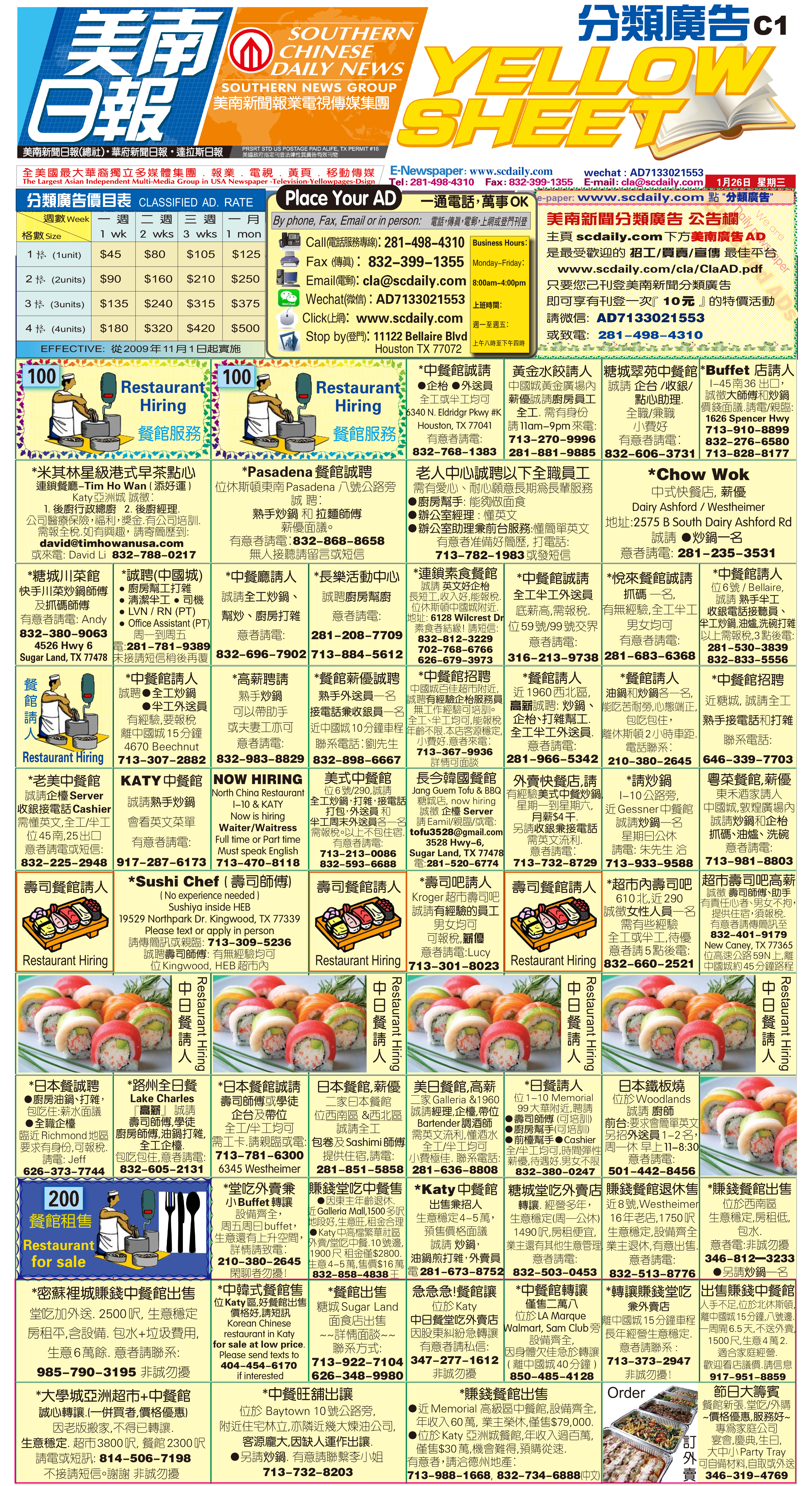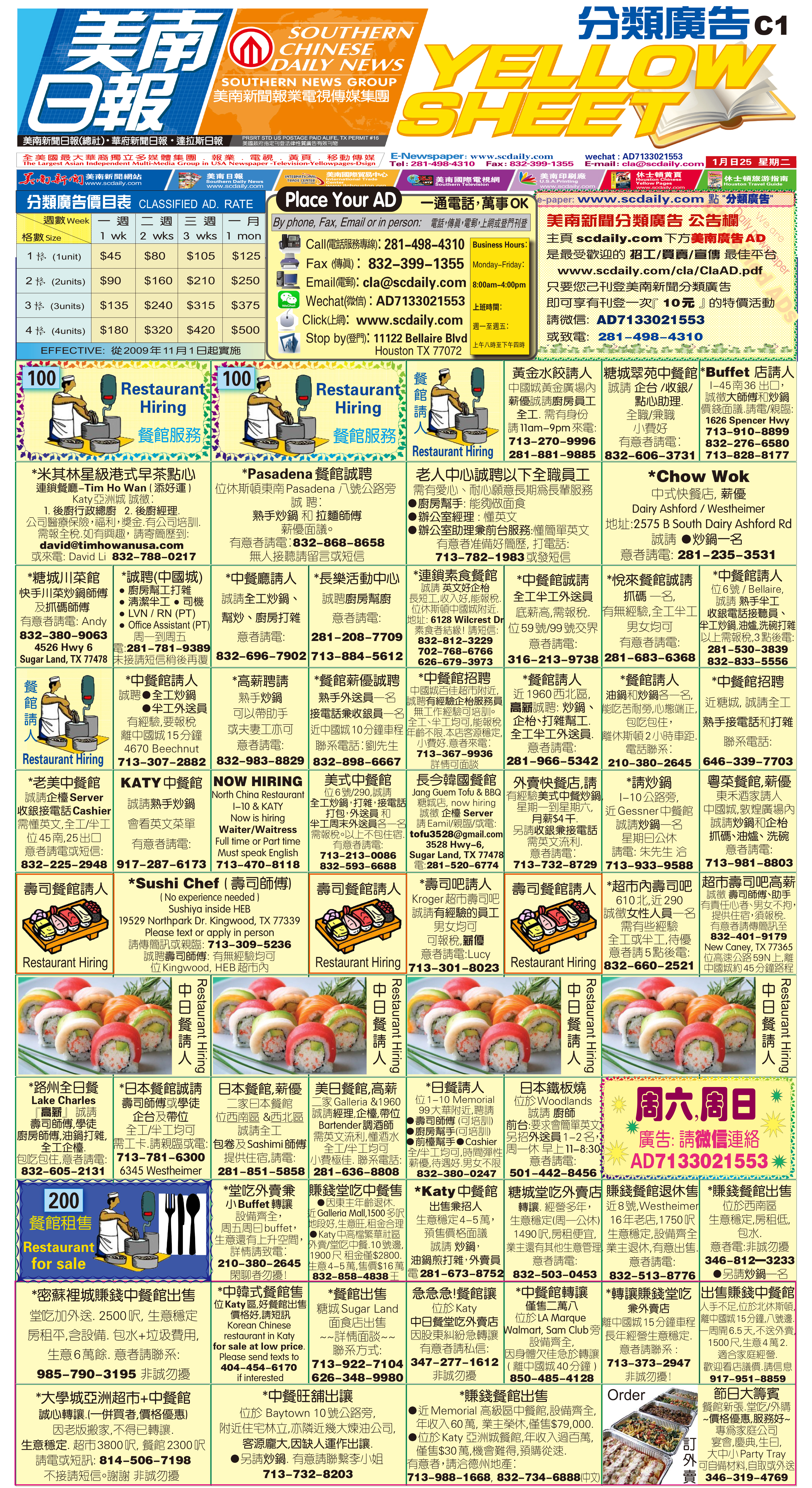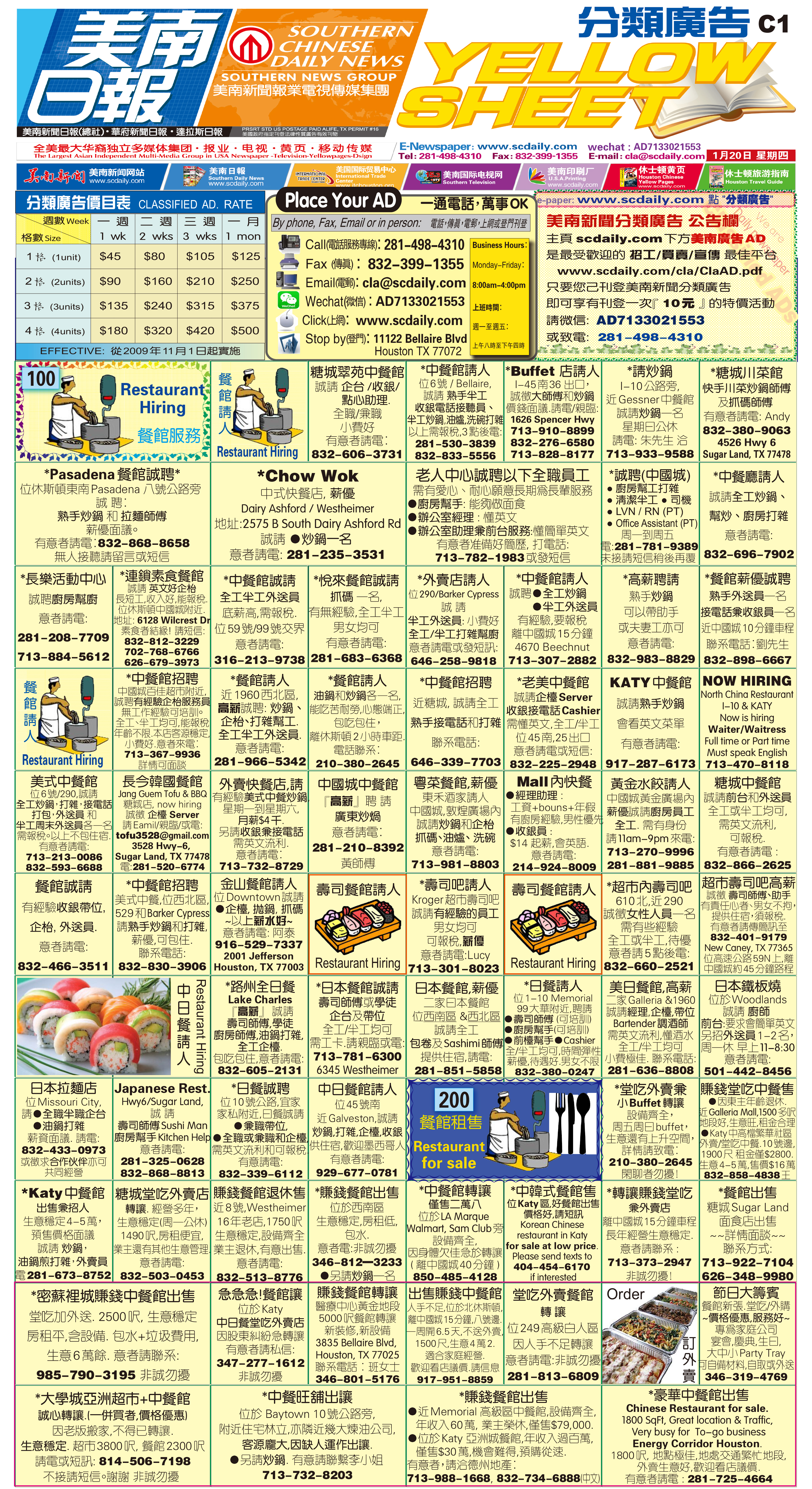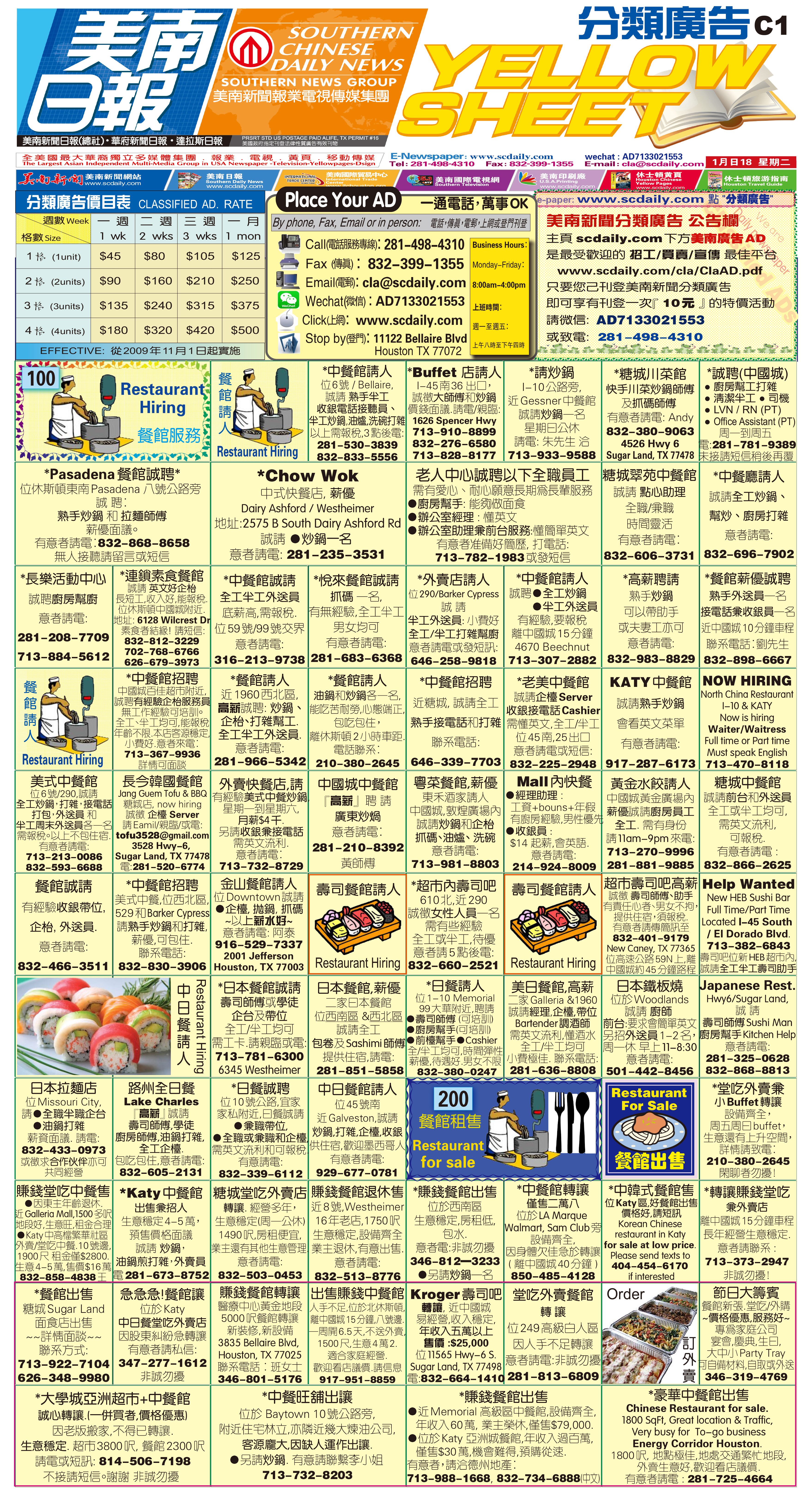211211 Epaper

 A Section B SectionC Section D Section220126 Epaper

 A Section B SectionC Section D Section220125 Epaper

 A Section B SectionC Section D Section220124 Epaper

 A Section B SectionC Section D Section220123 Epaper

 A Section B SectionC Section D Section220122 Epaper

 A Section B SectionC Section D Section220121 Epaper

 A Section B SectionC Section D Section220120 Epaper

 A Section B SectionC Section D Section220118 Epaper

 A Section B SectionC Section D Section220119 Epaper

 A Section B SectionC Section D Section220117 Epaper

 A Section B SectionC Section D Section